REPEATED TRIALS & EXPECTED VALUES

1. We are now going to start bringing together the concepts of probability and the components of an observed value.

1. Remember, we said that observed values or measurements or scores can be thought of as

observed score = true value + chance error + bias

1. The probability of an event occurring is a statement about the true possibility of an event, not about our observation itself

So if we were to flip a coin, we expect heads to occur with a probability of .5, or the coin should land as heads half the time. We may not see it.  It doesn't always occur, but that is our expectation

1. We can also imagine situations where we don't actually look at data we collect, but try to anticipate what data would like if we collected it.

Example: What would happen if we flipped a coin 100 times? How many times would we predict that heads would occur?

We can use the probability of an event to generate a hypothetical sampling distribution

Example: If the probability of heads as an outcome is .5, then if we flipped 100 times we should observe around 50 outcomes that are heads. Do we expect it to be exactly 50 every time we flip a coin 100 times?

1. The law of averages states that over repeated independent trials we will observe a value on average that is close to our expectation.  That is, the average of a large sample of independently selected observations from a random variable will approach the expected value (or mean) of the random variable distribution.

1. In statistics this is similar to the law of large numbers (as a sample drawn from a population gets so large as to be the population then the mean of the sample will have to be the mean of the population) .

2. The Law of Averages states, in the long run, over repeated trials, random fluctuations eventually average out and the average of our observations will approach the expected value. But at the same time with increasing numbers of observations, the number of observations that differ from what we expect will be larger.

1. Most people however believe in the law of small of numbers. We expect even short sequences of random events to show the kind of average behavior that in fact appears only in the long run.

1. Gambler's fallacy: Each event is independent. But in gamblers' fallacy we believe that because of an event occurring in one direction (like obtaining heads in a coin toss) then the next event must go in the opposite direction (tails). If they are independent, one trial has no effect on the other.

2. The belief influences our behavior (for example, pulling our bets off the table after 3 consecutive wins because we think our "luck" has run out).

3. Scientists, as people, are vulnerable to this also which is why we use statistics as a method to save us from our fallible judgment

1. To explore these issues further, we need to introduce the concept of a random variable

1. Definition: Let X represent a function that associates a real number with each and every elementary event in some sample space S. Then X is called a random variable on sample space S

2. Example: We can specify a random variable, x, which associates a number with all possible outcomes of the roll of a die. There are 6 elements in the sample space, or 6 possible outcomes that can occur.

 Number on die face 1 2 3 4 5 6 X 1 2 3 4 5 6

Example: Flips of a coin

 Coin flip outcomes Heads Tails X 0 1

To each event, we assign a value of x. The value that x takes on in any one roll or coin toss has a known probability. We can also sum the x values we obtain across events.

This is another way of saying that with repeated trials, we can create a sample of x values. Like all samples it will have a distribution that has both a center and a spread.

In Chapter 16, the authors give you some experience in creating samples of random variables.

1. You need to know which numbers go into the box

2. How many of each kind

3. How many draws

1. Random variables can be either discrete (there are only a finite number of possible outcomes) or continuous. In this class we will only deal with discrete random variables (continuous random variables require calculus).

1. Means of random variables

1. The expected value of a discrete random variable, X, denoted E(X) or µX is the long run average value for X.

The formula is: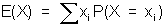For a coin toss: E(Heads)= 0*(0.5)+ 1 *(0.5) = 0.5

The expected value is found by multiplying each outcome by its probability and summing

1. Example: Let's say you play a shell game. If you pick the one with a coin under it you win \$10 on your bet of \$1. If you pick a shell without the coin, you lose \$5. How much can you expect to lose on each try on average?

If we do this as boxes, as in the book it looks like this:

1 chance of \$10 and 2 chances of -\$5

The average is (10 - 5 - 5)/3 = 0. This is a fair game.

With the formula, the answer is

E(X) = (10)(1/3) + (-5)(2/3) = 0

Notice that the \$ won or lost are xi and the P(X = xi) refers to the probability with winning or losing that amount

1. In the book, the authors refer to two types of expected values, only one of which is called an expected value

1. The expected winnings or loses on any one draw (average of the box)

2. The expected net gain, the total amount won or lost across draws

1. This is found by multiplying the number of draws times the average of the box

2. Example: if we played the shell 30 times, the net gain is 30 X \$0 = \$0.

1. Standard errors of random variables

1. Standard errors of random variables are the same idea as standard deviations of observed scores. SE's are the square root of the average of the squared deviations from the expected value, where in this case it is the expected value of the net gain. The expected value is center of the hypothetical sampling distribution, so it a mean. It is the center of what we would expect to observe if we in fact collected data. Standard deviations, remember, are the square root of the average of the squared deviations of observed scores from the mean.

2. In the book, SE refers to the standard error of the net gain and is calculated by:

SE = square root (number of draws) X (SD of the box)

The second part of this equation considers the average squared deviation from the mean expected in any given trial. The first part adjusts that for the sample size. Imagine you flipped a fair coin twice to count the number of heads. You expect in two flips to get one head. Your maximum expected standard deviation from the mean is small (less than a head); but if you flipped the sample coin 10,000 times you could have a large number of results that still centered around 5,000 heads.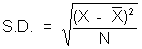Example: In the shell game: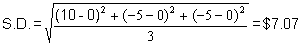If you played the game 30 times, then the S.E. would equal: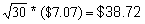1. The formula for the standard deviation of the box, which is also the standard deviation of a discrete random variable on any given try, can also be written: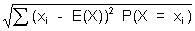or from our example: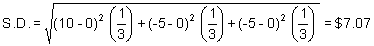1. The SE = square root (N) * S.D. = \$38.72